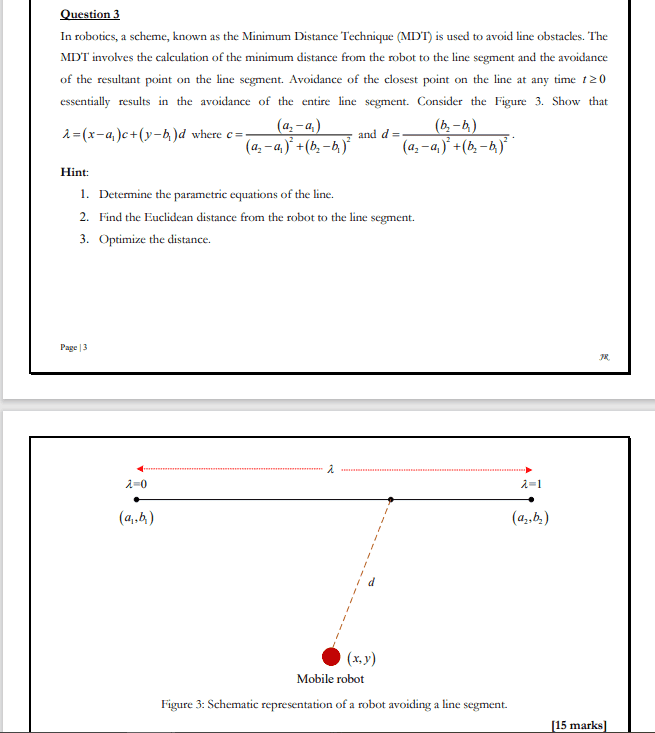# Parametric Equation and Euclidean Distance

• MHB
Aleister911HOI
Okay, what do you understand of this problem and what have you done on it? The first part asks for parametric equations for the line from $(x_1, y_1)$ to $(x_2, y_2)$. Do you know what "parametric equation" are? Do you know how to find parametric equations for a line?

Aleister911
I do understand that to find the parametric equation we focus on our vector equation which needs to be first computed (r=r0+tv). However there are no given values from each point to find r0 and tv to formulate the vector equation. I'm really confused with the overall idea of the question above. I kindly ask if anyone could help me solve this question.

HOI
You are told that the line passes through the points $(a_1, b_1)$ and $(a_2, b_2$.
A straight line can be written with parametric equations $x= r_1+ tv_1$ and and $y= r_2+ tv_2$. There are many different parametric equations describing the same line. We can arbitrarily take t= 0 at $(a_1, b_1)$ and t= 1 at $(a_2, b_2)$.

you need four equations to find the values of $r_1$, $v_1$, $r_2$, and $v_2$. Those four equation are:
$a_1= r_1+ 0v_1= r_1$
$b_1= r_2+ 0v_2= r_2$
$a_2= r_1+ 1v_1= r_1+ v_1$ and
$b_2= r_2+ 1v_2= r_2+ v_2$.

From the first two equations, $r_1= a_1$ and $r_2= a_2$. The next two equations give
$a_2= r_1+ v_1= a_1+ v_1$ so $v_1= a_2- a_1$ and $b_2= r_2+ v_2= a_2+ v_2$ so $v_2= b_2- a_2$

The parametric equations for the line are
$x= a_2+ (b_1- a_1)t$
$y= a_2+ (b_2- a_2)t$

Aleister911
Thank you for the respond. I do appreciate. My question is that are we going to use the parametric equation to solve for the euclidean distance from the robot to the line segment, using the euclidean formula D = sqrt[(x2-x1)^2+(y2-y1)^2]. If so then how can we determine the value of x1,x2,y1,y2 ??

HOI
Take any two points on the line. From a practical point of view, the farther the two points are, the more accurate the calculation will be so I would recommend the two endpoints of line, $(a_1, b_1)$ and $(a_2, b_2)$ in your picture.

Aleister911
Correct me if I'm wrong, but since we have 2 points, is it really mean that we should have two euclidean distance? if so then, can you help me solve this and optimise the distance as well?

Kind suggestion

Last edited:
HOI
No, you were not asked for the distance to the two endpoints. You were asked to find the distance from the point (x, y) to the line. That is the distance from (x, y) to the point at the foot of the perpendicular,

Aleister911
Okay, However from the Euclidean distance formula we have x2, x1 and y2,y1. What value are we going to used? is it the x,y values from the parametric equation?
I'm really confused now, can you help me solve this?

HOI
You use all the values! You are given two points, $(x_1, y_1)$ and $(x_2, y_2)$. Do you understand what $(x_1, y_1)$ and $(x_2, y_2)$ mean? Whoever gave you this problem expects you to be able to find the equation of the line between them. Can you do that?